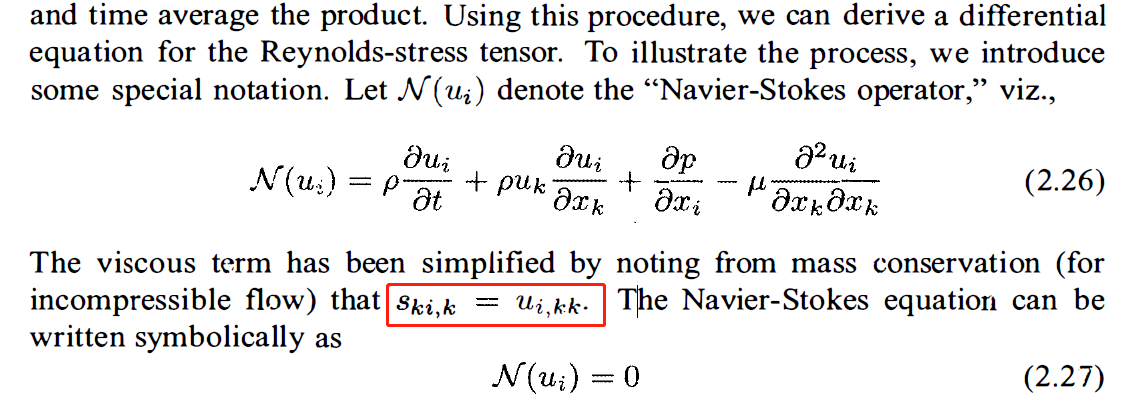# wilcox的turbulence教材中，某一步的导出的困惑。

• 各位大佬，在wilcox turbulence modeling for CFD 教材中，chapter 2.4 ，page -41中，如图所示，红框部分小弟理解不上去，请问书中这一步红线部分是从哪里来的，下标ki,k 和 i,kk代表什么，小弟没有理解上去，有没有大佬可以指点一二，浪费大家时间了，不胜感激。• 第一个下标表示分量，第二个下标表示导数。
\begin{equation}\notag
s_{12,1}=\frac{\p s_{12}}{\p x}=u_{2,11}=\frac{\p}{\p x}\left(\frac{\p u_2}{\p x}\right)
\end{equation}

• @李东岳 感谢李老师的回复，一下子就通透了